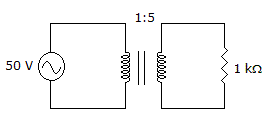# Electronics - Transformers

### Exercise :: Transformers - General Questions

11.

A special transformer used to convert unbalanced signals to balanced signals is the

 A. balun B. autotransformer C. center-tapped transformer D. step-across transformer

Explanation:

No answer description available for this question. Let us discuss.

12.If the load doubled in value in the given circuit, what reflected resistance would the source see?

 A. 80B. 400C. 2 kD. 10 kExplanation:

No answer description available for this question. Let us discuss.

13.

If the primary power of an ideal transformer having a 2:1 voltage ratio is 100 W, the secondary power is

 A. 100 W B. 50 W C. 75 W D. 200 W

Explanation:

No answer description available for this question. Let us discuss.

14.

A transformer has

 A. primary and secondary windings, both of which are considered inputs B. primary and secondary windings, both of which are considered outputs C. a primary winding used as an output and a secondary winding used as an input D. a primary winding used as an input and a secondary winding used as an output